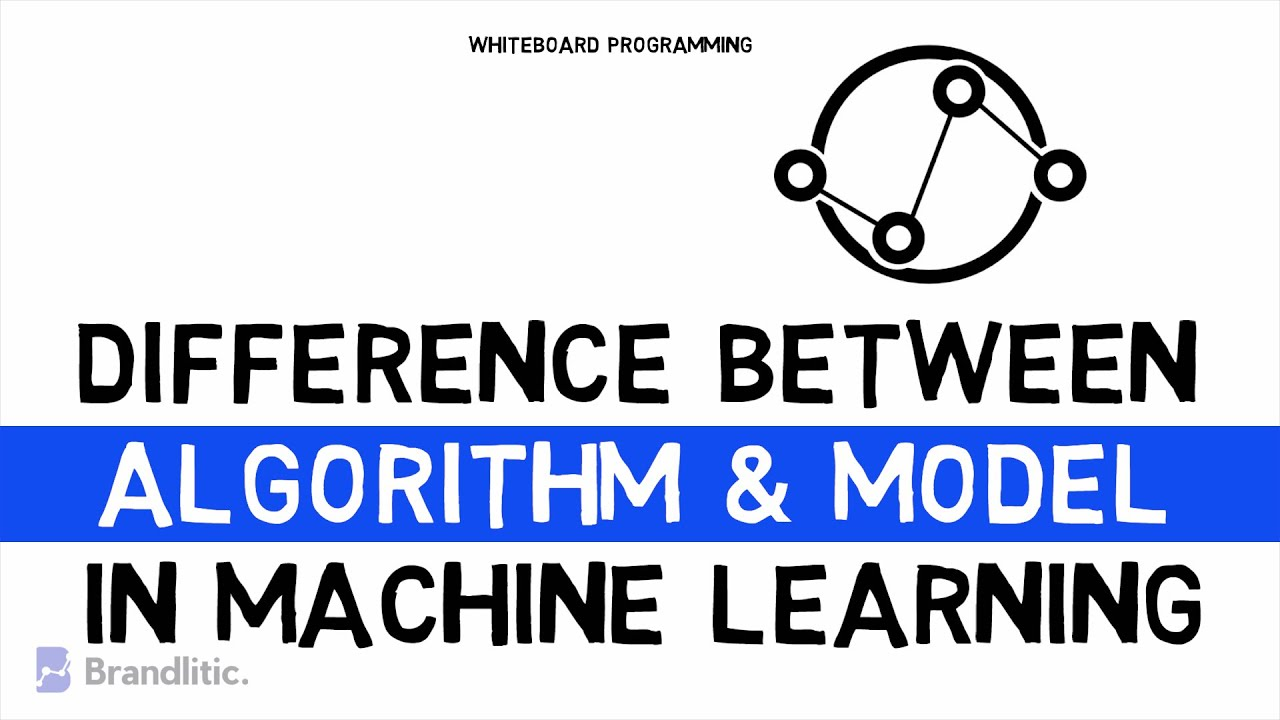1595227800

# Difference Between ML Algorithm and ML Model

ML Algorithm vs ML Model: Well, for a beginner, the words “algorithm" & "model” in machine learning creates a lot of confusion. Yes, there is a difference between machine learning algorithm and model. This video will help you understand each with a deeper insight and crucially understand which one to use as a term when.

After watching this video, you’ll be able to answer,

• What is an Algorithm in Machine Learning
• What is a Model in Machine Learning
• Algorithm vs. Model in ML
• Difference between Machine Learning Algorithm and Model

#machine-learning #developer

## Buddha Community1592318880

## Difference Between Algorithm and Model in Machine Learning

For beginners, this is very confusing as often “machine learning algorithm” is used interchangeably with “machine learning model.” Are they the same thing or something different?

As a developer, your intuition with “algorithms” like sort algorithms and search algorithms will help to clear up this confusion.

In this post, you will discover the difference between machine learning “algorithms” and “models.”

After reading this post, you will know:

• Machine learning algorithms are procedures that are implemented in code and are run on data.
• Machine learning models are output by algorithms and are comprised of model data and a prediction algorithm.
• Machine learning algorithms provide a type of automatic programming where machine learning models represent the program.

#machine learning #algorithm #model1595557020

## Difference Between ML Algorithm and ML Model

ML Algorithm vs ML Model: Well, for a beginner, the words “algorithm" & "model” in machine learning creates a lot of confusion. Yes, there is a difference between machine learning algorithm and model. This video will help you understand each with a deeper insight and crucially understand which one to use as a term when.

After watching this video, you’ll be able to answer,

• What is an Algorithm in Machine Learning
• What is a Model in Machine Learning
• Algorithm vs. Model in ML
• Difference between Machine Learning Algorithm and Model

#algorithms1626872040

## What Is Model & Algorithm In Machine Learning | Machine Learning Tutorials | Python 04

What Is Model & Algorithm In Machine Learning | Machine Learning Tutorials | Python | Ml Python

#python #machine learning #algorithm #model & algorithm #machine learning tutorials1617366480

## Shortcut Learning: The Reason ML Models Often Fail in Practice

TLDR, models always take the route of least effort.

Training machine learning models is far from easy. In fact, the unaware data scientist might trip and fall in as many pitfalls as there are living AWS instances. The list is endless but divides itself nicely into two broad categories: underfitting, your model is bad, and overfitting, your model is still bad, but you think it isn’t. While overfitting can manifest itself in various ways, shortcut learning is a recurring flavor when dealing with custom datasets and novel problems. It affected me; it might be affecting you.

Informally, shortcut learning occurs whenever a model fits a problem on data not expected to be relevant or present, in general.

#ai & machine learning #algorithms #machine learning #ml models #shortcut learning1595296029

## Deploying trained ML model on Heroku using Flask | End-to-End ML Project Tutorial

The series will cover everything from Data Collection to Model Deployment using Flask Web framework on Heroku!

GitHub Repository: https://github.com/dswh/fuel-consumpt…

#ml #heroku #ml #deploying